﻿ 大当量TNT空中爆炸超压的模拟与修正 Simulation and Correction of Large Equivalent TNT Air Explosion Overpressure

International Journal of Mechanics Research
Vol. 08  No. 04 ( 2019 ), Article ID: 33316 , 9 pages
10.12677/IJM.2019.84026

Simulation and Correction of Large Equivalent TNT Air Explosion Overpressure

Ya Wang, Hong Zhang, Xiang Chen

School of Science, Chang’an University, Xi’an Shaanxi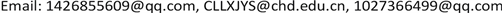Received: Nov. 14th, 2019; accepted: Nov. 28th, 2019; published: Dec. 5th, 2019ABSTRACT

In order to study the explosive characteristics of large equivalent explosives, the LS-DYNA software was used to establish a model for the explosion of large equivalent TNT explosives in air. The calculation result is lower than the empirical formula value and can be used as the lower limit of anti-explosion design. Through the simulation values of different working conditions, the general overpressure function is obtained by fitting, and the numerical simulation results can be directly calculated. The overpressure function is corrected in the form of exponential function, and the universal modified overpressure function is given, which avoids the calculation deviation caused by different empirical formulas. Through data verification, both functions have universal applicability, which can better guide the structural anti-explosion design of large equivalent explosives and facilitate engineering application.

Keywords:Explosion, Numerical Simulation, Large Equivalent, Overpressure Correction1. 引言

2. 空气冲击波传播规律

3. 数值模拟和经验公式的对比

3.1. 建立爆炸模型和材料本构关系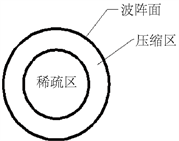Figure 1. Spherical explosive shock wave propagation diagram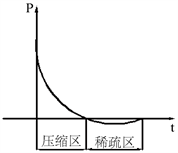Figure 2. Overpressure decay law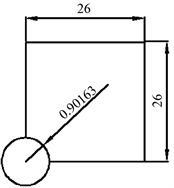Figure 3. Model plane sketch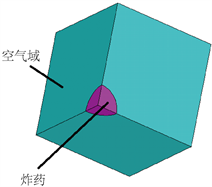Figure 4. Entity model diagram

$\begin{array}{l}P={C}_{0}+{C}_{1}\mu +{C}_{2}{\mu }^{2}+{C}_{3}{\mu }^{3}+\left({C}_{4}+{C}_{5}\mu +{C}_{6}{\mu }^{2}\right)E,\text{\hspace{0.17em}}\\ \text{\hspace{0.17em}}\mu =\frac{1}{V}-1\end{array}$ (1)

$P=A\left(1-\frac{\omega }{{R}_{1}V}\right){\text{e}}^{-{R}_{1}V}+B\left(1-\frac{\omega }{{R}_{2}V}\right){\text{e}}^{-{R}_{2}V}+\frac{\omega E}{V}$ (2)Table 2. Air material parameters and equation of state parametersTable 3. Explosive material parameters and equation of state parameters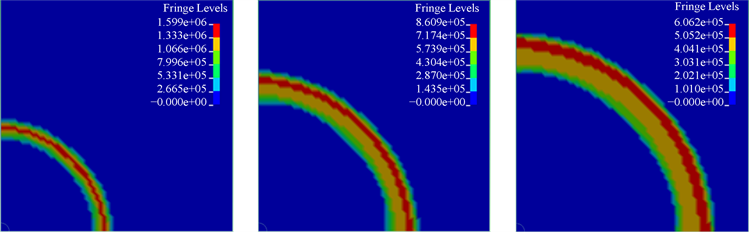(a) t = 0.005 s (b) t = 0.01 s (c) t = 0.015 s

Figure 5. Overpressure cloud example

3.2. 经验公式

$P=\left\{\begin{array}{l}\frac{1.07}{{Z}^{3}}-0.1\text{}Z\le 1.0\\ \frac{0.076}{Z}+\frac{0.255}{{Z}^{2}}+\frac{0.65}{{Z}^{3}}\text{}1.0 (3)

2) Henrych公式在1979提出的计算超压峰值的表达式   ：

$P=\left\{\begin{array}{l}\frac{1.40717}{Z}+\frac{0.55397}{{Z}^{2}}-\frac{0.03572}{{Z}^{3}}+\frac{0.000625}{{Z}^{4}}\text{}0.05\le Z\le 0.3\text{}\\ \frac{0.61938}{Z}-\frac{0.03262}{{Z}^{2}}+\frac{0.21324}{{Z}^{3}}\text{}0.3\le Z\le 1\\ \frac{0.0662}{Z}+\frac{0.405}{{Z}^{2}}+\frac{0.3288}{{Z}^{3}}\text{}1\le Z\le 10\end{array}$ (4)

3) Mills公式在1987年提出的计算TNT爆炸的超压峰值的方法   ：

$P=\frac{0.108}{Z}-\frac{0.114}{{Z}^{2}}+\frac{1.772}{{Z}^{3}}$ (5)

4) 我国国防工程设计规范(草案)中规定的空爆冲击波超压公式为  ：

$P=\frac{0.084}{Z}+\frac{0.27}{{Z}^{2}}+\frac{0.7}{{Z}^{3}}$ (6)

5) 王儒策(1993)根据原子爆炸的经验装药提出在无限空气介质中爆炸的超压公式  ：

$P=\frac{0.082}{Z}+\frac{0.26}{{Z}^{2}}+\frac{0.69}{{Z}^{3}}$ (7)

6) 人民防空地下室设计规范(GB50038-2005)  中提到的公式为：

$P=\frac{1.316}{{Z}^{3}}+\frac{0.369}{{Z}^{1.5}}$ (8)

3.3. 结果误差对比Figure 6. Six formula overpressure peak curve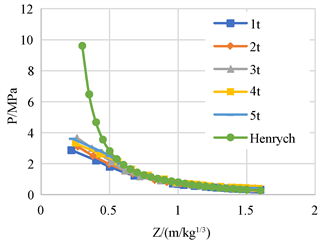Figure 7. Overpressure peak curve of different equivalent TNT and Henrych formulas

3.4. 结果修正

1) 根据最小二乘法，运用Matlab软件，取5种当量下的超压峰值平均值，拟合得到数值模拟通用超压函数表达式，如式(2.9)：

$\Delta P=\left\{\begin{array}{l}\frac{0.1613}{Z}+\frac{0.7746}{{Z}^{2}}-\frac{0.1639}{{Z}^{3}}\text{}0.3\le Z\le 1.0\\ -\frac{0.0441}{Z}+\frac{1.2772}{{Z}^{2}}-\frac{0.4964}{{Z}^{3}}\text{}1.0\le Z\le 1.6\end{array}$ (9)

${P}^{\prime }=\eta \Delta P$ (10)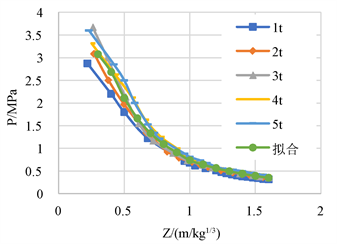Figure 8. Comparison of simulation results and fitting results

2) 为了验证式(2.9)和(2.12)的可靠性，建立6 t的炸药模型，拟合得到图9的对比曲线，结果可见：(a) 公式(2.9)可以很好的反映模拟结果，说明该函数具有普遍适用性；(b) 利用公式(2.12)修正后的结果与经验公式平均值吻合程度较高，说明了该修正系数函数是可靠的。

3) 为了验证式(2.9)和(2.12)的可靠性，建立6 t的炸药模型，拟合得到图9的对比曲线，结果可见：(a) 公式(2.9)可以很好的反映模拟结果,说明该函数具有普遍适用性；(b) 利用公式(2.12)修正后的结果与经验公式平均值吻合程度较高，说明了该修正系数函数是可靠的。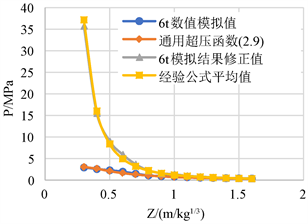Figure 9. Contrast curve

4) 综上所述，修正后的冲击波通用超压函数表达式可归纳如式(2.13)。为了在实际中应用方便，直接采用式(2.9)计算数值模拟值，避免繁琐的分析过程及对人员素质要求过高等问题；以式(2.13)计算修正后的超压值，避免采用不同经验公式计算引起的偏差。

${P}^{\prime }=\left\{\begin{array}{l}\left(\frac{0.1613}{Z}+\frac{0.7746}{{Z}^{2}}-\frac{0.1639}{{Z}^{3}}\right)\left(380{\text{e}}^{-13.17Z}+7.899{\text{e}}^{-1.686Z}\right),\text{}0.3\le Z\le 1.0\\ \left(-\frac{0.0441}{Z}+\frac{1.2772}{{Z}^{2}}-\frac{0.4964}{{Z}^{3}}\right)\left(14.92{\text{e}}^{-3.324Z}+1.4011{\text{e}}^{-0.2283Z}\right),\text{}1.0 (13)

4. 结论

1) 不同的经验公式因为提出的背景、环境有差别，数据之间的差距较大，其中Mills公式值最大，Henrych公式值最小。

2) 运用LS-DYNA软件建立大当量TNT炸药在空气中爆炸的模型，得出数值模拟值比经验公式值小，如果仅以模拟解进行结构抗爆设计，存在低估爆炸威力的风险，但是可作为抗爆设计的下限参考值。

3) 通过不同工况的模拟值，拟合得到通用超压函数表达式(2.9)，可以直接计算数值模拟结果，避免工程应用中繁琐的建模分析过程及对人员素质要求过高等问题；通过修正模拟值，归纳出通用修正超压函数表达式(2.13)，该公式避免了不同经验公式计算引起的偏差，更好的指导结构针对大当量炸药的抗爆设计。

Simulation and Correction of Large Equivalent TNT Air Explosion Overpressure[J]. 力学研究, 2019, 08(04): 229-237. https://doi.org/10.12677/IJM.2019.84026

1. 1. 李忠献, 任其武, 师燕超, 等. 重要建筑结构抗恐怖爆炸设计爆炸荷载取值探讨[J]. 建筑结构学报, 2016, 37(3): 51-58.

2. 2. 李宝岩, 戴智涵. 大当量TNT自由空气爆炸数值模拟研究[C]//第26届全国结构工程学术会议论文集(第III册). 北京: 工程力学杂志社, 2017: 96-100.

3. 3. 石少卿, 康建功, 汪敏, 等. ANSYS/LS-DYNA在爆炸与冲击领域内的工程应用[M]. 北京: 中国建筑工业出版社, 2011: 18-22.

4. 4. 李翼祺, 马素贞. 爆炸力学[M]. 北京: 科学出版社, 1959: 259.

5. 5. 李晓勇, 崔村燕, 陈景鹏. LS-DYNA软件开展爆炸冲击波计算时需考虑的问题[J]. 装备学院学报, 2014(4): 79-84.

6. 6. (2006) LS-DYNA Keyword User’s Manual. Livermore California: Liver-more Software Technology, Corporation.

7. 7. Larcher, M. (2007) Simulation of the Effects of an Air Blast Wave. JRC Technical Note, Institute for the Protection and Security of the Citizen.

8. 8. Zhou, T., Yuan, B.-H. and Liang, Z.-F. (2006) Experimental Study of Jet Initiation of Shield PBX. Chinese Journal of Explosives & Propellants, 29, 10-13.

9. 9. 吴媛媛, 宋振森. 挡墙后爆炸冲击波绕流现象研究[J]. 河北工程大学学报(自然科学版), 2014, 31(2): 9-14.

10. 10. 亨利奇. 爆炸动力学及其应用[M]. 熊建国, 译. 北京: 科学出版社, 1987: 127.

11. 11. 杨鑫, 石少鑫, 程鹏飞. 空气中TNT爆炸冲击波超压峰值的预测及其数值模拟[J]. 爆破, 2008, 25(1): 15-19.

12. 12. Tolba, A.F.F. (2001) Response of FRP-Retrofitted Reinforced Concrete Panels to Blast Loading. Canada: Carleton University.

13. 13. 王儒策, 赵国志. 弹丸终点效应[M]. 北京: 北京理工大学出版社, 1993: 45.

14. 14. 中华人民共和国建设部. GB50038-2005. 人民防空地下室设计规范[S]. 北京: 中国计划出版社, 2004.